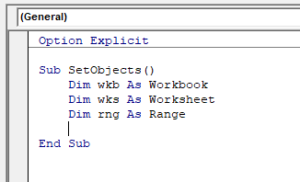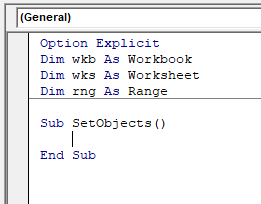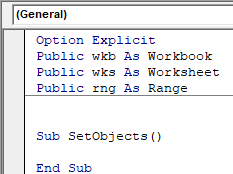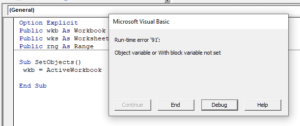# VBA Set Object Variables – Workbooks, Worksheets, & More

This tutorial will teach you how to define object variables using the Set statement in VBA.

## Defining Object Variables

Objects are the cornerstone of Microsoft Office – without objects, we cannot achieve anything.  In Excel, objects include the Workbook, Worksheet or Range Objects. In Microsoft Word, examples are the Document or Table object. Each object has a variety of Properties and Methods that can be programmed to control the behavior of that object.

## Declaring the Object Variable

Before we can reference the object in code, and therefore control the object, we need to declare the object. We can do this using the Dim Statement.

``````Dim wkb as Workbook
Dim wks as Worksheet
Dim Rng as Range
Dim wdDoc as Document
Dim wdTbl as Table
Dim shp as Shape``````

This Dim declaration can occur inside a procedure:or outside a procedure at the module-level:If the variable is declared at the module-level (outside the procedure), the variable can be used throughout the module.

If the object variable is declared with the Public statement then the variable can be used throughout the VBA Project:## Set Value

Once you have declared the object, you need to assign a value to the object. This must be done using the Set statement and can only be done within a Procedure.

``````Sub SetObjects()
Set wkb = ActiveWorkbook
Set wks = Sheet1
Set rng = Range("A1:G4")
End Sub
``````

Note: This is different than assigning values to non-object variables. You MUST use the Set statement to assign the object to the variable. If you do not, you’ll receive an error:Once you have assigned a value to the object, you can then write code to control the behavior or manipulate the object.

## Object Examples in Excel

### Workbook Object

Once you have declared a workbook variable, you can then assign a workbook to that object and use the Properties and Methods available to manipulate that object. In the example below we are going to save a workbook.

``````Sub WorkbookObject()
'declare the workbook object
Dim wkb as Workbook
'assign an unsaved workbook to the object
Set wkb = Workbooks("Book1")
'save the workbook
wkb.SaveAs "C:\data\testbook.xlsx"
'close the workbook
wkb.close
'remember to release the object
Set wkb = Nothing
End Sub
``````

### Worksheet Object

Similarly you can manipulate a worksheet or worksheets once you have declared the worksheet as a variable. In the example below, we rename Sheet1 and Sheet2.

``````Sub WorksheetObject()
Dim wks1 As Worksheet
Dim wks2 As Worksheet
'initialize the objects
Set wks1 = Sheet1
Set wks2 = Sheet2
'rename the sheets
wks1.Name = "Customers"
wks2.Name = "Products"
'set the objects to nothing
Set wks1 = Nothing
Set wks2 = Nothing
End Sub``````

### Range Object

The Range object is one of the most  useful objects to manipulate in Excel. In the example below, we bold Range A1 to E1 and format it with a bottom border.

``````Sub RangeObject()
Dim rng1 As Range
'intialize the range
Set rng = Range("A1:E1")
'bold the range and set the bottom border
rng.Font.Bold = True
With rng1.Borders(xlEdgeBottom)
.LineStyle = xlContinuous
.ColorIndex = 0
.Weight = xlThin
End With
End Sub``````

### Shape Object

You can also use object variables to work with shapes.

``````Sub AddShape()
Dim shp As Shape
'create the shape
Set shp = ActiveSheet.Shapes.AddShape(msoShapeSmileyFace, 68.25, 225.75, 136.5, 96#)
With shp
'change inside colour and style
.Fill.ForeColor.RGB = RGB(255, 255, 0)
.Fill.Solid